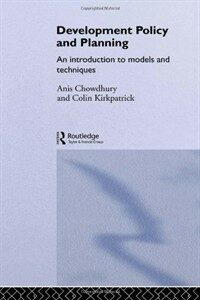> 상세정보

# 상세정보## Development policy and planning : an introduction to models and techniques (2회 대출)

자료유형
단행본
개인저자
Chowdhury, Anis, 1954- Kirkpatrick, C. H. (Colin H.), 1944-.
서명 / 저자사항
Development policy and planning : an introduction to models and techniques / Anis Chowdhury and Colin Kirkpatrick.
발행사항
London ;   New York :   Routledge,   1994.
형태사항
xii, 132 p. : ill. ; 23 cm.
ISBN
0415098882 0415098890 (pbk.)
서지주기
Includes bibliography (p. 123-126) and index.
일반주제명
Economic development --Mathematical models. Economic policy --Mathematical models. Planning --Developing countries --Mathematical models.
 000 00983camuuu200277 a 4500 001 000000900949 005 19990115142126.0 008 930304s1994 enka b 001 0 eng 010 ▼a 93007404 020 ▼a 0415098882 020 ▼a 0415098890 (pbk.) 040 ▼a DLC ▼c DLC ▼d DLC ▼d 244002 043 ▼a d------ 049 0 ▼l 151011544 050 0 0 ▼a HD75.5 ▼b .C52 1994 082 0 0 ▼a 338.9/001/5118 ▼2 20 090 ▼a 338.9001 ▼b C552d 100 1 ▼a Chowdhury, Anis, ▼d 1954- 245 1 0 ▼a Development policy and planning : ▼b an introduction to models and techniques / ▼c Anis Chowdhury and Colin Kirkpatrick. 260 ▼a London ; ▼a New York : ▼b Routledge, ▼c 1994. 300 ▼a xii, 132 p. : ▼b ill. ; ▼c 23 cm. 504 ▼a Includes bibliography (p. 123-126) and index. 650 0 ▼a Economic development ▼x Mathematical models. 650 0 ▼a Economic policy ▼x Mathematical models. 650 0 ▼a Planning ▼z Developing countries ▼x Mathematical models. 700 1 ▼a Kirkpatrick, C. H. ▼q (Colin H.), ▼d 1944-.

### 소장정보

No. 소장처 청구기호 등록번호 도서상태 반납예정일 예약 서비스
No. 1 소장처 청구기호 338.9001 C552d 등록번호 151011544 도서상태 대출가능 반납예정일 예약 서비스

### 컨텐츠정보

#### 목차

```
CONTENTS
List of figures = ⅸ
List of tables = ⅹ
Preface = xi
1 DEVELOPMENT POLICY ANALYSIS AND QUANTITATIVE PLANNING METHODS = 1
Development policy and planning: an overview = 1
Characteristics of development planning models = 4
2 AGGREGATE CONSISTENCY MODELS = 12
The Harrod-Domar growth model = 12
The two-gap model = 17
3 DISAGGREGATED CONSISTENCY MODELS = 22
Introduction = 22
Main-sector models = 22
Multi-sector models: input-output analysis = 28
Policy applications of input-output analysis = 35
Extending the model = 44
Appendix: Essentials of matrix algebra = 46
4 MULTI-SECTORAL MODELS AND THE SOCIAL ACCOUNTING MATRIX = 58
Introduction = 58
The social accounting matrix = 58
Limitations of multi-sectoral planning models = 63
5 PROGRAMMING APPROACH TO PLANNING = 66
Introduction = 66
Linear programming = 69
Shadow pricing of resources = 77
Economic interpretation of dual solutions = 79
Appendix: The simplex method = 81
Appendix: Sensitivity analysis = 87
6 COMPUTABLE GENERAL EQUILIBRIUM MODELS = 94
Introduction = 94
The CGE model approach = 95
An illustrative CGE model = 99
Uses of CGE models = 107
7 COST-BENEFIT ANALYSIS = 109
Introduction = 109
Financial project appraisal = 110
Economic project appraisal = 113
The social discount rate = 118
Distributional issues = 119
Policy analysis and cost-benefit analysis = 121
Bibliography = 123
Index = 127

```

### 관련분야 신착자료

#### The Market power of technology : understanding the second gilded age

Kurz, Mordecai (2023)

#### (2023) 디지털 트윈 기술개발과 응용, 서비스 사업화 전략 : AI, IoT, 메타버스로 구현하는 DX(디지털전환)

CHO Alliance (2023)

양승룡 (2023)

#### 디지털 팩토리 : 디지털 자본주의 시대, 보이지 않는 노동

Altenried, Moritz (2023)

이현경 (2023)

김용원 (2023)

정수환 (2022)

한국맥도날드 (2023)

SK 네트웍스 (2023)

정운찬 (2023)

권오상 (2023)

문경연 (2023)

이은석 (2022)

김진우 (2022)

박의연 (2022)

박현 (2023)

국립민속박물관 (2023)